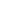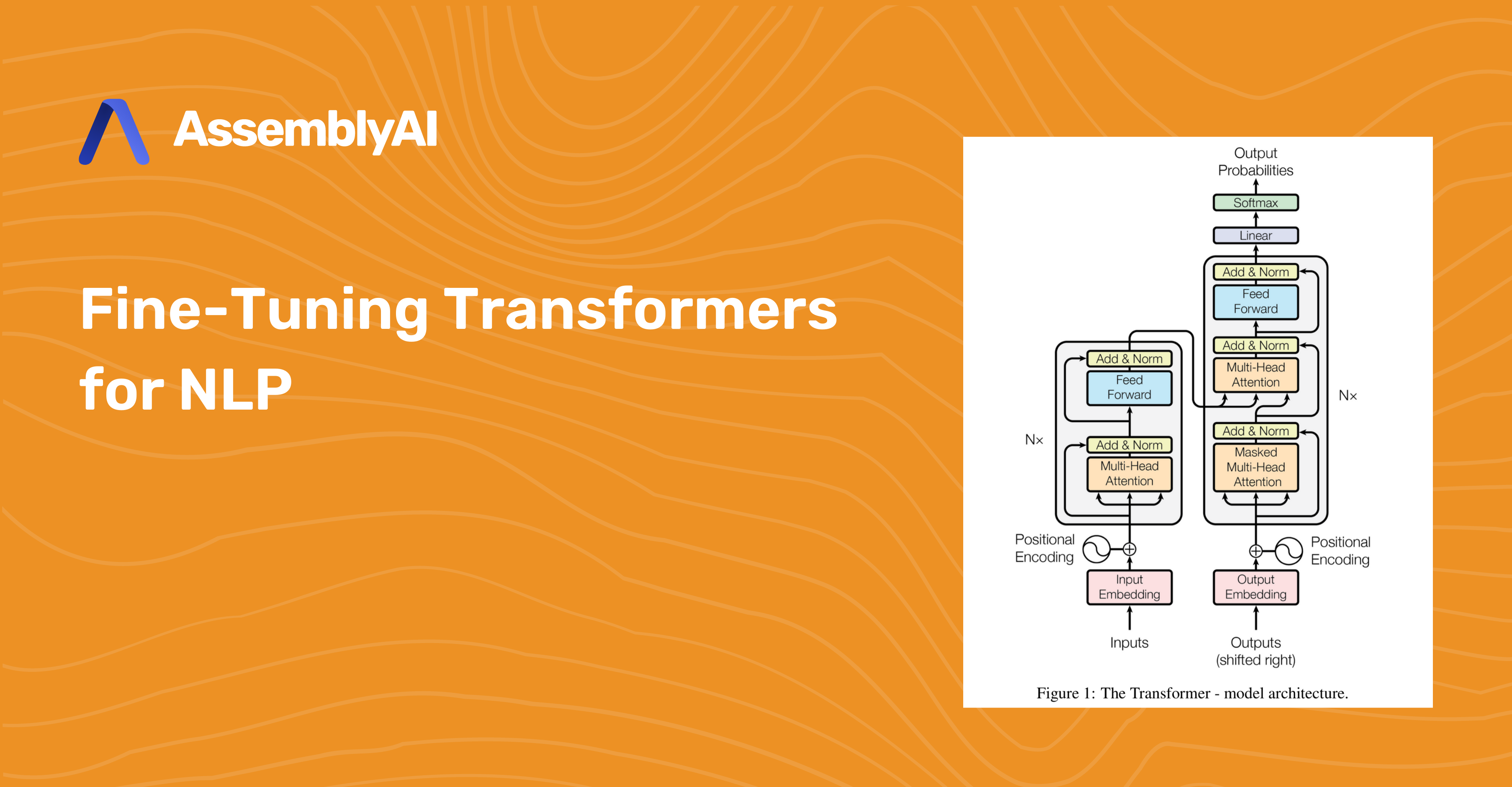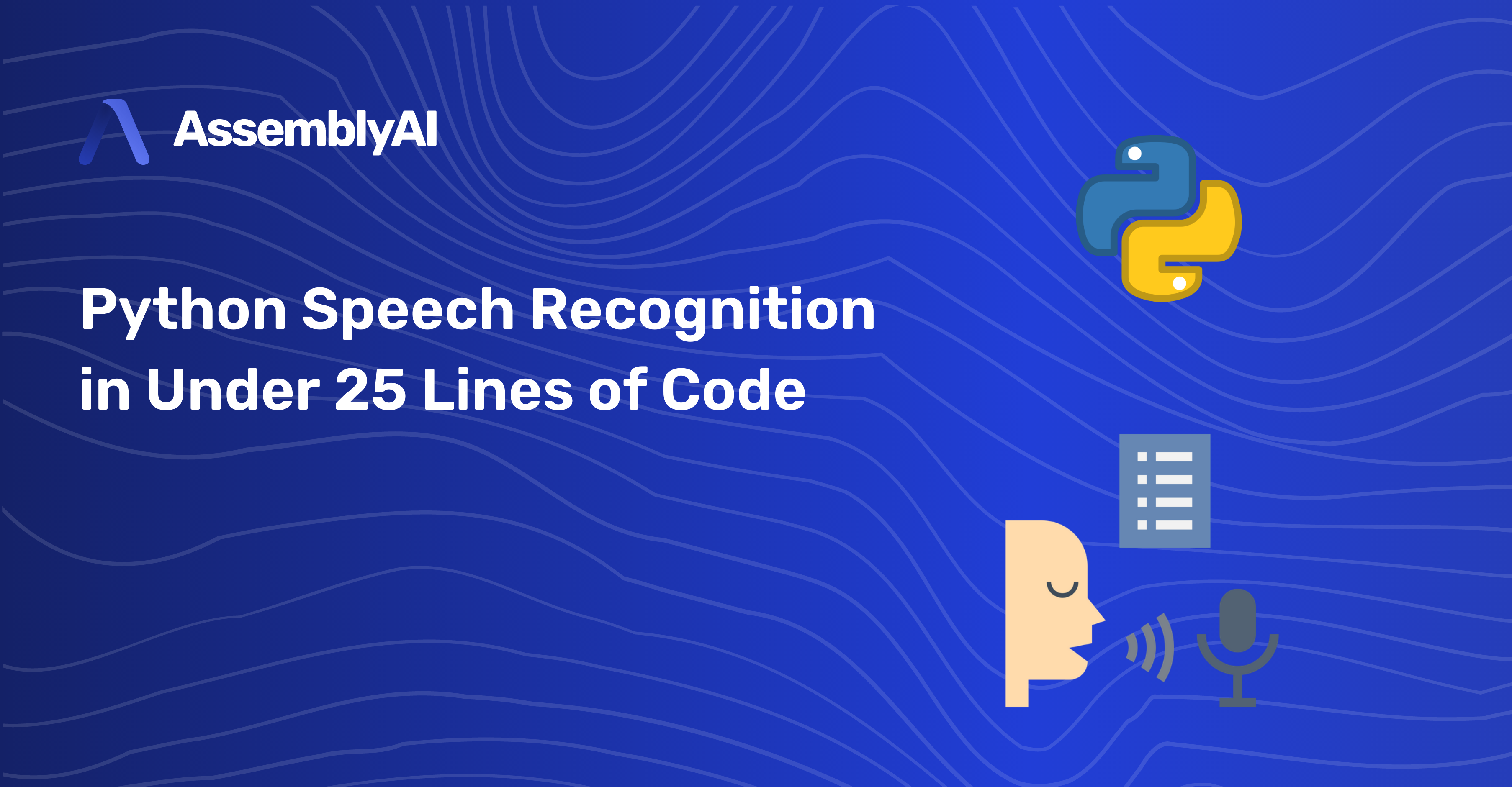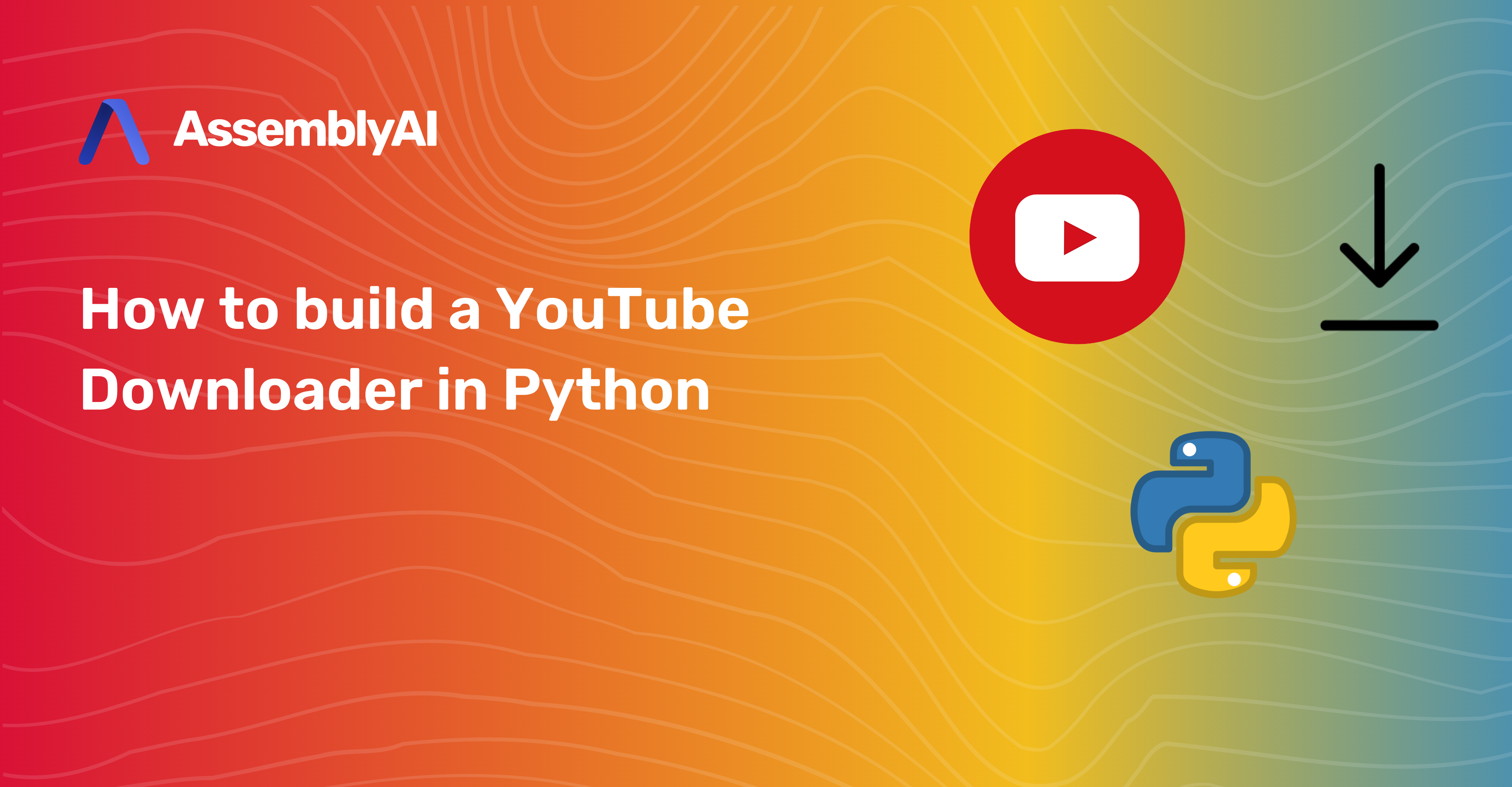Blog

# Fine-Tuning Transformers for NLP

Deep Learning
TutorialsIn this tutorial, we'll show how you to fine-tune two different transformer models, BERT and DistilBERT, for two different NLP problems: Sentiment Analysis, and Duplicate Question Detection.

You can see a complete working example in our Colab Notebook, and you can play with the trained models on HuggingFace. Let's jump in!

### Intro

Since being first developed and released in the Attention Is All You Need paper Transformers have completely redefined the field of Natural Language Processing (NLP) setting the state-of-the-art on numerous tasks such as question answering, language generation, and named-entity recognition. Here we won't go into too much detail about what a Transformer is, but rather how to apply and train them to help achieve some task at hand. The main things to keep in mind conceptually about Transformers are that they are really good at dealing with sequential data (text, speech, etc.), they act as an encoder-decoder framework where data is mapped to some representational space by the encoder before then being mapped to the output by way of the decoder, and they scale incredibly well to parallel processing hardware (GPUs).

Transformers in the field of Natural Language Processing have been trained on massive amounts of text data which allow them to understand both the syntax and semantics of a language very well. For example, the original GPT model published in Improving Language Understanding by Generative Pre-Training was trained on BooksCorpus, over 7,000 unique unpublished books. Likewise, the famous BERT model released in the paper BERT: Pre-training of Deep Bidirectional Transformers for Language Understanding was trained on both BooksCorpus and English Wikipedia. For readers interested in diving into the neural network architecture of a Transformer, the original paper and The Illustrated Transformer are two great resources.

The main benefit behind Transformers, and what we will take a look at throughout the rest of this blog, is that once pre-trained Transformers can be quickly fine-tuned for numerous downstream tasks and often perform really well out of the box. This is primarily due to the fact that the Transformer already understands language which allows training to focus on learning how to do question answering, language generation, named-entity recognition, or whatever other goal someone has in mind for their model.

### Datasets

#### Stanford Sentiment Treebank v2 (SST2)

The first task models will be trained for is sentiment analysis. Sentiment analysis is a long-standing benchmark in the field of NLP with the goal in mind to be able to detect whether some text is positive, negative, or somewhere in between. This has many use cases such as detecting if a product is viewed in a positive or negative manner based on customer reviews or if a candidate has a high or low approval rating based on tweets. The dataset we will use to train a sentiment analysis model is the Stanford Sentiment Treebank v2 (SST2) dataset which contains 11,855 movie review sentences. This task and dataset is part of the General Language Understanding Evaluation (GLUE) Benchmark which is a collection of resources for training, evaluating, and analyzing natural language understanding systems.

Here are some examples from this dataset where numbers closer to 0 represent negative sentiment and numbers closer to 1 represent positive:

Sentence Label
The Rock is destined to be the 21st Century's new "Conan" and that he's going to make a splash even greater than Arnold Schwarzenegger, Jean-Claud Van Damme or Steven Segal. 0.6944400072097778
The gorgeously elaborate continuation of "The Lord of the Rings" trilogy is so huge that a column of words can not adequately describe co-writer/director Peter Jackson's expanded vision of J.R.R. Tolkien's Middle-earth. 0.833329975605011
A thunderous ride at first, quiet cadences of pure finesse are few and far between; their shortage dilutes the potency of otherwise respectable action. 0.44444000720977783

#### Quora Question Pairs (QQP)

The second task models will be trained for is duplicate question detection. Likewise, this task also has various use cases such as removing similar questions from the Quora platform to limit confusion amongst users. The dataset we will use to train a duplicate question detection model is the Quora Question Pairs dataset. This task/dataset is also part of the GLUE Benchmark.

A number of examples from this dataset where 0 represents non-duplicates and 1 represents duplicates are:

Question 1 Question 2 Label
How do I make money flying my drone? How can I use a dji phantom to make money 1
What can you do with an economics degree? What jobs can you get with an economics degree? 1
What type of current does a battery produce? How does a generator work and produce current? 0

### Models

Two different Transformer based architectures will be trained for the tasks/datasets above. Pre-trained models will be loaded from the HuggingFace Transformers Repo which contains over 60 different network types. The HuggingFace Model Hub is also a great resource which contains over 10,000 different pre-trained Transformers on a wide variety of tasks.

#### DistilBERT

The first architecture we will train is DistilBERT which was open sourced and released in DistilBERT, a distilled version of BERT: smaller, faster, cheaper, and lighter. This Transformer is 40% smaller than BERT while retaining 97% of the language understanding capabilities and also being 60% faster. We will train this architecture for both the SST2 and QQP datasets.

#### BERT

The second architecture we will train is BERT published in BERT: Pre-training of Deep Bidirectional Transformers for Language Understanding. This was the first Transformer that really showed the power of this model type in the NLP domain by setting a new state-of-the-art on eleven different NLP tasks at the time of its release.

We will train this architecture for the SST2 dataset only.

### Fine-Tuning

With this background in mind let's now take a look at the code and train/fine-tune these models! Here we use the PyTorch deep learning framework and only include code for the SST2 dataset. To run this code yourself feel free to check out our Colab Notebook which can be easily edited to accomodate the QQP dataset as well.

#### Creating the Dataset

First let's create our PyTorch Dataset class for SST2. This class defines three important functions with the following purposes:

• __init__: initializes the class and loads in the dataset
• __len__: gets the length of the dataset
• __getitem__: selects a random item from the dataset
```#Libraries needed
import torch
from torch.utils.data import Dataset

#PyTorch dataset class
class  SST_Dataset(Dataset):
#Name: 		__init__
#Purpose: 	init function to load the dataset
#Inputs: 	dataset -> dataset
#Outputs: 	none
def  __init__(self, dataset):
self.dataset = dataset
return

#Name: 		__len__
#Purpose: 	get the length of the dataset
#Inputs: 	none
#Outputs: 	length -> length of the dataset
def  __len__(self):
return  len(self.dataset)

#Name: 		__getitem__
#Purpose: 	get a random text segment and its label from the dataset
#Inputs: 	idx -> index of the random text segment to load
#Outputs: 	text -> text segment
# 			label -> sentiment score
def  __getitem__(self, idx):
text =  self.dataset[idx]['sentence']
label = torch.zeros(2)
label[round(self.dataset[idx]['label'])] =  1
return text, label
```

#### Helper Functions

Next let's create a couple helper functions to do things like get the GPU, transfer data to it, etc. Neural networks, especially Transformer based ones, nearly always train faster on accelerator hardware such as GPUs so it is critical to send both the model and data there for processing if it's available. This allows for a significant training speedup as parallel processing capabilities can be utilized.

```#Name: 		get_gpu
#Purpose: 	checks if a GPU device is avaliable
#Input: 	none
#Output: 	GPU -> GPU device if applicable, none if not
def  get_gpu():
#Check if a GPU is avaliable and if so return it
GPU  =  None
if torch.cuda.is_available():
print("Using GPU")
GPU  = torch.device("cuda")
else:
print("No GPU device avaliable! Using CPU")
return  GPU

#Name: 		transfer_device
#Purpose: 	transfers model / data to the GPU devie if present
#Inputs: 	GPU -> GPU device if applicable, none if not
# 		 	data -> data to transfer
#Output: 	data -> data that has been transferred if applicable
def  transfer_device(GPU, data):
if(GPU  !=  None):
data = data.to(GPU)
return data

#Name: 		count_correct
#Purpose: 	count the number of correct model predictions in a batch
#Inputs: 	predictions -> model predictions
#		 	targets -> target labels
#Outputs: 	correct -> number of correct model predictions
def  count_correct(predictions, targets):
#Create variables to store the number of correct predictions along with the index of the prediction in the batch
correct =  0
index =  0

#Loop across all predictions in the batch and count the number correct
while(index <  len(predictions)):
#Convert the prediction and target to lists
prediction =  list(predictions[index])
target =  list(targets[index])

#Get the max index indicating the truth value from the prediction and target
prediction_index = prediction.index(max(prediction))
target_index = target.index(max(target))

#If the max indices are the same increment correct
if(prediction_index == target_index):
correct +=  1
index +=  1
return correct
```

#### Defining the Loss Function

Now we will define the loss function... Since we are training a classifier to predict whether a sentence has positive or negative sentiment, or if two questions are duplicates, we will use the binary cross entropy loss function. The math behind this loss is:

Here y is the true label (0 or 1) whereas p(y) is our model prediction. Through the minimization of this value our network learns to make more accurate predictions.

```#Name: 		binary_cross_entropy
#Purpose: 	defines binary cross entropy loss function
#Inputs: 	predictions -> model predictions
# 			targets -> target labels
#Outputs: 	loss -> loss value
def  binary_cross_entropy(predictions, targets):
loss =  -(targets * torch.log(predictions) + (1  - targets) * torch.log(1  - predictions))
loss = torch.mean(loss)
return loss
```

#### Model Training / Evaluation

Next lets write the core training/evaluation logic to fine-tune and test our model which consists of 3 primary functions:

• train_model
• train
• evaluate

The train_model function works by first evaluating the pre-trained model on the validation set and calculating the performance before any training has taken place. This function then loops over three epochs while training the model on the training set and evaluating its performance on the validation set. An epoch is essentially a loop over all the data in some dataset.

The train function operates by training the model for an epoch. Note that before any training takes place our model is put into training mode indicating to PyTorch that gradients need to be stored for parameter updates. All batches in an epoch are then looped over by iterating over the PyTorch Dataloader. Each batch is then passed through the tokenizer allowing these tokens to then be sent to the model for sentiment score predictions. Following the de facto PyTorch training loop setup, a loss value is computed, the optimizer is zeroed out, gradients are derived on the loss, and the model is updated by taking an optimizer step.

The evaluate function has a similar setup to train except the final optimizer zeroing out, gradient derivation, and optimizer step are removed since the model should not be trained on a validation set. Other differences between these two functions is that here our model is set to evaluation mode which allows for faster inference since gradients don't need to be stored.

Built into both the train and evaluate function is a call to count_correct which computes the number of correct sentiment score predictions per batch allowing a final accuracy score to be derived across the entire dataset. Also note that softmax is called over the model's output mapping scores to probabilities.

```import torch.nn.functional as F

#Name: 		train_model
#Purpose: 	train the model while evaluating its performance
#Inputs: 	GPU -> GPU device to train / evaluate on
# 			tokenizer -> text tokenizer for model
# 			model -> model to train / evaluate
# 			optimizer -> optimizer to use to update model parameters
# 			criterion -> criterion to use to compute loss values
#Outputs: 	model -> model after training
#Evaluate the performance of the model before training
valid_loss, valid_accuracy = evaluate(GPU, dev_dataloader, tokenizer, model, criterion)
print("Pre-training validation loss: "+str(valid_loss)+" --- Accuracy: "+str(valid_accuracy))
print()

#Train the model across 3 epochs and evaluate its performance
for epoch in  range(3):
model, train_loss, train_accuracy = train(GPU, train_dataloader, tokenizer, model, optimizer, criterion)
valid_loss, valid_accuracy = evaluate(GPU, dev_dataloader, tokenizer, model, criterion)

#Print performance stats
print(" ", end="\r")
print("Epoch: "+str(epoch+1))
print("Training loss: "+str(train_loss)+" --- Accuracy: "+str(train_accuracy))
print("Validation loss: "+str(valid_loss)+" --- Accuracy: "+str(valid_accuracy))
print()
return model
```
```#Name: 		train
#Purpose: 	train the model over 1 epoch
#Inputs: 	GPU -> GPU device to train on
# 			tokenizer -> text tokenizer for model
# 			model -> model to train
# 			optimizer -> optimizer to use to update model parameters
# 			criterion -> criterion to use to compute loss values
#Outputs: 	model -> model after training over the epoch
# 			average_loss -> average loss over the epoch
# 			accuracy -> accuracy over the epoch
def  train(GPU, dataloader, tokenizer, model, optimizer, criterion):
#Place the network in training mode, create a variable to store the total loss, and create a variable to store the total number of correct predictions
model.train()
total_loss =  0
total_correct =  0

#Loop through all batches in the dataloader
for batch_number, (texts, labels) in  enumerate(dataloader):
#Tokenize the text segments, get the model predictions, compute the loss, and add the loss to the total loss
tokenized_segments = tokenizer(texts, return_tensors="pt", padding=True, truncation=True)
loss = criterion(model_predictions, transfer_device(GPU, labels))
total_loss += loss.item()

#Count the number of correct predictions by the model in the batch and add this to the total correct
correct = count_correct(model_predictions.cpu().detach().numpy(), labels.numpy())
total_correct += correct

#Zero the optimizer, compute the gradients, and update the model parameters
loss.backward()
optimizer.step()

#Compute the average loss and accuracy across the epoch
return model, average_loss, accuracy
```
```#Name: 		evaluate
#Purpose: 	evaluate the model over 1 epoch
#Inputs: 	GPU -> GPU device to evaluate on
# 			tokenizer -> text tokenizer for model
# 			model -> model to evaluate
# 			criterion -> criterion to use to compute loss values
#Outputs: 	average_loss -> average loss over the epoch
# 			accuracy -> accuracy over the epoch
def  evaluate(GPU, dataloader, tokenizer, model, criterion):
#Place the network in evaluation mode, create a variable to store the total loss, and create a variable to store the total number of correct predictions
model.eval()
total_loss =  0
total_correct =  0

#Loop through all batches in the dataloader
for batch_number, (texts, labels) in  enumerate(dataloader):
#Tokenize the text segments, get the model predictions, compute the loss, and add the loss to the total loss
tokenized_segments = tokenizer(texts, return_tensors="pt", padding=True, truncation=True)
loss = criterion(model_predictions, transfer_device(GPU, labels))
total_loss += loss.item()

#Count the number of correct predictions by the model in the batch and add this to the total correct
correct = count_correct(model_predictions.cpu().detach().numpy(), labels.numpy())
total_correct += correct

#Compute the average loss and accuracy across the epoch
return average_loss, accuracy
```

### Putting it all Together

Now that we have defined all the functions needed to train our model, we can finally fine-tune it and see what happens! Note that SST2 is one of many dataset stored in HuggingFace Datasets making it incredibly easy to load and use.

```from datasets import load_dataset
from transformers import DistilBertTokenizer, DistilBertForSequenceClassification

#Get the GPU device if it exists, load the SST-2 dataset, and create PyTorch datasets and dataloaders for the training and validation sets
GPU  = get_gpu()
train_dataset = SST_Dataset(sst2_dataset['train'])
valid_dataset = SST_Dataset(sst2_dataset['validation'])

#Create the tokenizer, model, optimizer, and criterion
tokenizer = DistilBertTokenizer.from_pretrained('distilbert-base-uncased')
model = transfer_device(GPU, DistilBertForSequenceClassification.from_pretrained('distilbert-base-uncased'))
criterion = binary_cross_entropy

#Train and save the model
torch.save({
'tokenizer': tokenizer,
'model_state_dict': model.state_dict()},
model+".pt")
return
```

To train a BERT model instead of DistilBERT use the following:

```from transformers import BertTokenizer, BertForSequenceClassification
tokenizer = BertTokenizer.from_pretrained('bert-large-uncased')
model = transfer_device(GPU, DistilBertForSequenceClassification.from_pretrained('distilbert-base-uncased'))
```

### Results

#### SST2

After fine-tuning both DistilBERT and BERT on the SST2 dataset for 3 epochs their performance was evaluated on the validation and test sets. Numbers below are accuracy scores averaged across 8 separate model training runs:

Dataset DistilBERT BERT
Validation 83.992% 87.443%
Test 85.056% 86.997%

#### QQP

Although code for training on QQP is not shown in this blog, our Colab Notebook can easily be modified to accomodate this data. The primary changes to make are editing the PyTorch dataset to handle two text inputs to a model, question 1 and question 2, as well as adjusting the input to the tokenizer. Results from fine-tuning DistilBERT on QQP for 3 epochs with performance being evaluated on the validation set can be seen below. Note that the accuracy score is averaged across 8 separate model training runs:

Dataset DistilBERT
Validation 89.909%

### Conclusion

In this blog we learned how to fine-tune Transformers on downstream tasks, specifically sentiment analysis and duplicate question detection. By fine-tuning pre-trained Transformers significant time can be saved with performance often immediately high out of the box. This is in comparison to training from scratch which takes longer and uses orders of magnitude more compute and energy to reach the same performance metrics.

Feel free to check out the Colab Notebook that comes with this blog to run the experiments yourself! Also, if you would like to download and use the models we have developed they can be found on the HuggingFace Model Hub at the following locations:

### Subscribe to our blog!

Oops! Something went wrong while submitting the form.

## You may also like

Checkout some of our recent research and product updatesTutorials
Python Speech Recognition in Under 25 Lines of CodeTutorials